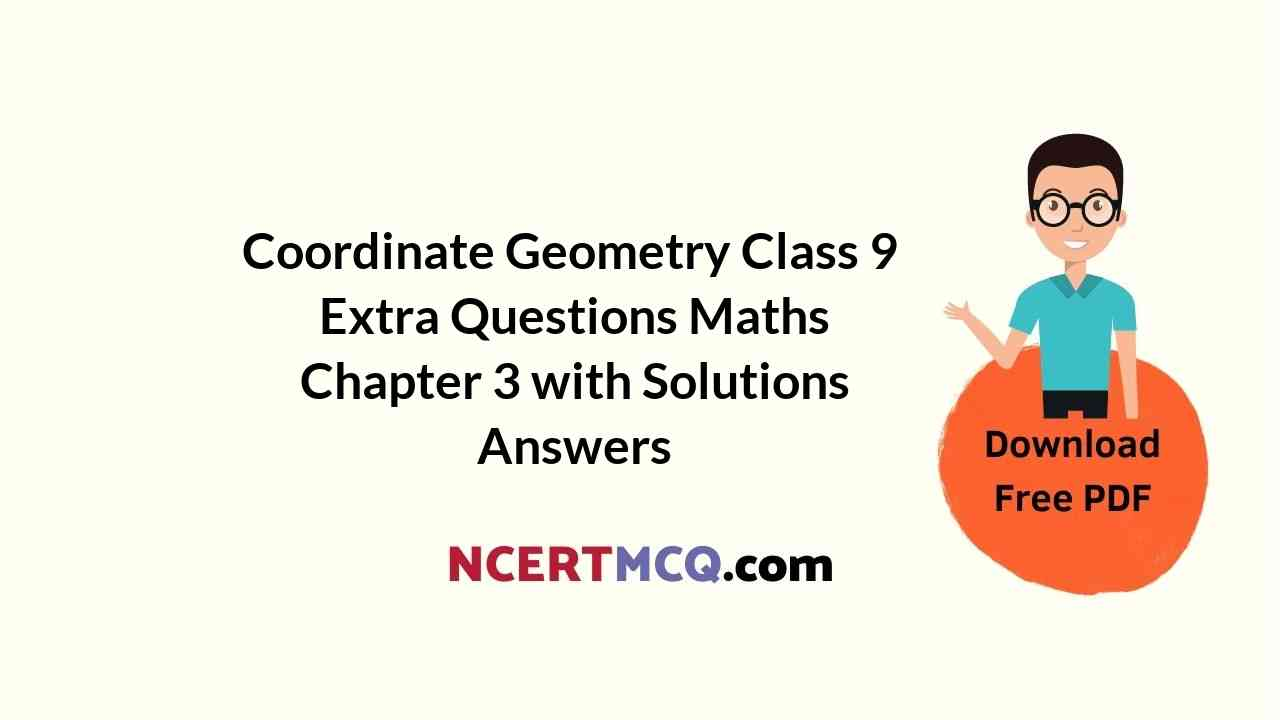Here we are providing Coordinate Geometry Class 9 Extra Questions Maths Chapter 3 with Answers Solutions, Extra Questions for Class 9 Maths was designed by subject expert teachers. https://ncertmcq.com/extra-questions-for-class-9-maths/

## Extra Questions for Class 9 Maths Coordinate Geometry with Answers Solutions

Extra Questions for Class 9 Maths Chapter 3 Coordinate Geometry with Solutions Answers

### Coordinate Geometry Class 9 Extra Questions Very Short Answer Type

Coordinate Geometry Class 9 Extra Questions Question 1.
Write the signs convention of the coordinates of a point in the second quadrant.
Solution:
(-ve, +ve)

Class 9 Coordinate Geometry Extra Questions Question 2.
Write the value of ordinate of all the points lie on x-axis.
Solution:
0

Coordinate Geometry Extra Questions Class 9 Question 3.
Write the value of abscissa of all the points lie on y-axis.
Solution:
0

Class 9 Maths Chapter 3 Extra Questions Question 4.
If in coordinates of a point B(3, -2), signs of both coordinates are interchanged, then it will lie in which quadrant ?
Solution:
When signs of both coordinates of B(3, -2) are interchanged, then coordinates of new point are B'(-3, 2) and it will lie in second quadrant.

Extra Questions On Coordinate Geometry Class 9 Question 5.
Find distances of points C(-3, -2) and D(5, 2) from x-axis and y-axis.
Solution:
Distances of point C(-3, -2) from x-axis is 2 units in the negative direction and from y-axis is 3 units in the negative direction. Distances of point D(5, 2) from x-axis is 2 units and from y-axis is 5 units.

Extra Questions Of Coordinate Geometry Class 9 Question 6.
Find the values of x and y, if two ordered pairs (x – 3, – 6) and (4, x + y) are equal.
Solution:
Here, two ordered pairs are equal.
⇒ Their first components are equal and their second components are separately equal.
⇒ x – 3 = 4 and x + y = -6
⇒ x = 7 and 7 + y = -6 ⇒ y = – 13
Hence, x = 7 and y = – 13.

Questions On Coordinate Geometry Class 9 Question 7.
In which quadrant does the point (-1, 2) lie ?
Solution:
(-1, 2) lie in second quadrant.

Coordinate Geometry Class 9 Important Questions Question 8.
Find the distance of the point (0, -5) from the origin.
Solution:
5 units.

Class 9 Maths Coordinate Geometry Extra Questions Question 9.
Write the shape of the quadrilateral formed by joining (1, 1), (6, 1), (4, 5) and (3, 5) on graph paper.
Solution:
Trapezium.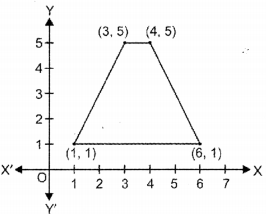### Coordinate Geometry Class 9 Extra Questions Short Answer Type 1

Coordinate Geometry Class 9 Questions With Solutions Question 1.
In the given figure, ABCD is a rectangle with length 6 cm and breadth 3 cm. O is the mid-point of AB. Find the coordinates of A, B, C and D.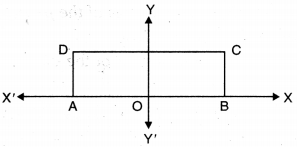Solution: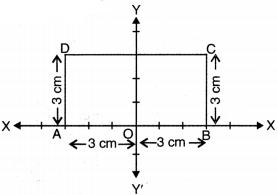We have taken 1 cm = 1 unit and origin O is the mid-point of AB
∴ OA = OB = 3 cm
and BC = AD = 3 cm
Thus, the coordinates of A are (-3, 0)
the coordinates of B are (3, 0)
the coordinates of C are (3, 3)
the coordinates of D are (-3, 3)

Ch 3 Maths Class 9 Extra Questions Question 2.
Write the coordinates of A, B, C and D from the figure given alongside.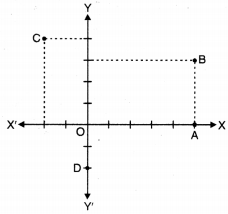Solution:
Coordinates of the point A are (5, 0)
Coordinates of the point B are (5, 3)
Coordinates of the point C are (-2, 4)
Coordinates of the point D are (0, -2)

Class 9 Maths Ch 3 Extra Questions Question 3.
A point lies on x-axis at a distance of 9 units from y-axis. What are its coordinates? What will be the coordinates of a point, if it lies on y-axis at a distance of -9 units from x-axis ?
Solution:
As shown in graph, the coordinates of a point which lies on x-axis at a distance of 9 units from y-axis are (9, 0) and the coordinates of a point which lies at a distance of -9 units from x-axis are (0, -9).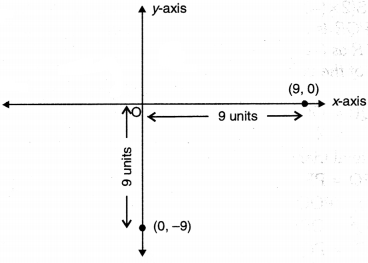Coordinate Geometry Class 9 Hots Questions Question 4.
Plot the point P(2, -6) on a graph paper and from it draw PM and PN perpendiculars to x-axis and y-axis respectively. Write the coordinates of the points M and N.
Solution: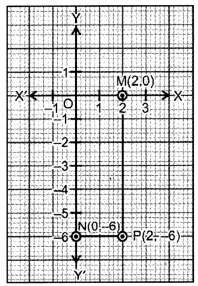As shown in graph, coordinates of M are (2, 0) and coordinates of N are (0, -6).

### Coordinate Geometry Class 9 Extra Questions Short Answer Type 2

Chapter 3 Maths Class 9 Extra Questions Question 1.
Without plotting the points indicate the quadrant in which they lie, if :
(i) ordinate is 5 and abscissa is – 3
(ii) abscissa is -5 and ordinate is – 3
(iii) abscissa is – 5 and ordinate is 3
(iv) ordinate is 5 and abscissa is 3
Solution:
(i) Clearly, point (-3, 5) lies in 2nd quadrant.
(ii) Clearly, point (-5, – 3) lies in 3rd quadrant.
(ii) Clearly, point (-5, 3) lies in 2nd quadrant.
(iv) Clearly, point (3, 5) lies in 1st quadrant.

Coordinate Geometry Class 9 Extra Questions With Solutions Question 2.
Plot the points A(1, 4), B(-2, 1) and C(4, 1). Name the figure so obtained on joining them in order and also, find its area.
Solution: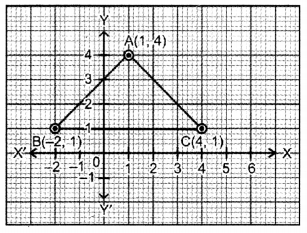Triangle.
Area of ∆ABC = $$\frac{1}{2}$$ × BC × Height
= $$\frac{1}{2}$$ × 6 × 3
= 9 sq. units

Coordinate Geometry Questions Class 9 Question 3.
Plot the following points, join them in order and identify the figure thus formed : A(1, 3), B(1, -1), C(7, -1) and D(7, 3)
Write the coordinates of the point of intersection of the diagonals.
Solution: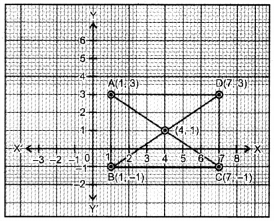ABCD is a rectangle.
Point of intersection of the diagonals AC and BD is (4, 1).

Class 9 Chapter 3 Maths Extra Questions Question 4.
Plot the points A(2, 5), B(8,5) and C(5, -3) and join AB, BC and CA. What figure do you obtain ?
Solution:We obtain an isosceles triangle in which AC = BC.

Extra Questions For Class 9 Maths Chapter 3 With Solution Question 5.
(i) Plot the points M(4, 3), N(4, 0), 0(0, 0), P(0, 3).
(ii) Name the figure obtained by joining MNOP.
(iii) Find the perimeter of the figure.
Solution:
(i)(ii) As shown in graph, the figure obtained by joining MNOP is rectangle.
(iii) Perimeter of rectangle MNOP = 2 (ON + OP) = 2 (4 + 3) = 2 × 7 = 14 units.

Question 6.
Plot D(-2, -3) on the graph paper. Also, plot reflections of D in x-axis and y-axis.
Solution: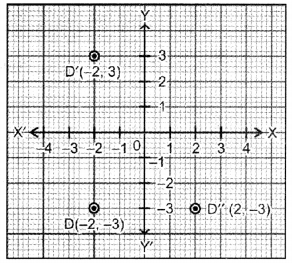Reflection of D(-2, -3) in x-axis is D'(-2, 3) and reflection of D(-2, -3) in y-axis is D'(2, -3).

Question 7.
If the coordinates of a point M are (-2, 9) which can also be expressed as (1 + x, y) and y > 0, then find in which quadrant do the following points lie : Ply, x), Q(2, x), R(x, y − 1), S(2x, -3y).
Solution:
Here, M(-2, 9) can also be expressed as (1 + x, y2)
∴ (1 + x, y2) ⇔ (-2, 9) and y > 0
⇒ 1 + x = -2 and y2 = 9
⇒ x = -3 and y = √9 = 3 (∵ y > 0)
P (y, x) = P(3, -3), it lies in IV quadrant
Q(2, x) = Q(2, -3), also lies in IV quadrant
R(x2, y – 1) = R((-3)2, 3 – 1) = R(9, 2), it lies in I quadrant
S(2x, -3y) = S(2 × (-3), -3 × 3) = S(-6, -9), it lies in III quadrant

Question 8.
In the given figure, PQR is an equilateral triangle with coordinates of Q and R as (-2, 0) and (2, 0) respectively. Find the coordinates of the vertex P.
Solution: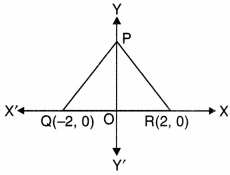Here,
QR = OQ + OR.
= 2 + 2 = 4 units
∴ ∆PQR is an equilateral triangle.
∴ PQ = PR = QR = 4 units
In right-angled ∆OPQ, ∠POQ = 90°
∴ We have PQ2 = OP2 + OQ2
⇒ OP2 = PQ2 – OQ2
= 42 – 22 = 16 – 4 = 12
⇒ OP = √12 = 2√3 units
∴ The coordinates of P are (0, 2√3).

### Coordinate Geometry Class 9 Extra Questions HOTS

Question 1.
Plot the points A(3, 2), B(-2, 2), C(-2, -2) and D(3, -2) in the cartesian plane. Join these points and name the figure so formed.
Solution:Figure so formed is ABCD a rectangle.

Question 2.
Write the coordinates of two points on X-axis and two points on Y-axis which are at equal distances from the origin. Connect all these points and make them as vertices of quadrilateral. Name the quadrilateral thus formed.
Solution:
Let a be the equal distance from origin on both axes. Now, the coordinates of two points on equal distance ‘a’on x-axis are Pla, 0) and R(-a, 0). Also, the coordinates of two points on equal distance ‘a’ on Y-axis are Q(0, a) and S(0, -a). Join all the four points on the graph. Now, PQRS, thus formed is a square.### Coordinate Geometry Class 9 Extra Questions Value Based (VBQs)

Question 1.
On environment day, class-9 students got five plants of mango, silver oak, orange, banyan and amla from soil department. Students planted the plants and noted their locations as (x, y).Plot the points (x, y) in the graph and join them in the given order. Name the figure you get. Which social act is being done by students of class-9 ?
Solution: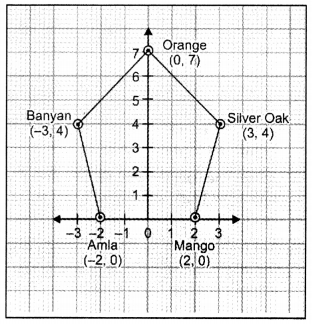The given trees (points) are Mango (2, 0), Silver Oak (3, 4), Orange (0,7), Banyan (-3, 4) and Amla (-2, 0). The location of these trees are Orange (0,7) shown in the graph.
On joining the points of mango, silver oak, orange, banyan and amla in order, the figure so formed is a regular pentagon.
Planting more trees helpful in reducing pollution and make the environment clean and green for the coming generations.